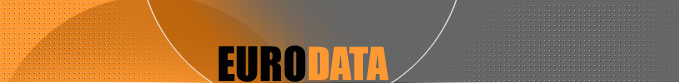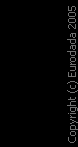05.08.2020HomeSite mapContacts# Validating Money Strings

Validating Money Strings
Ok, we can now use what we've learned to work on something useful: a regular expression to check user input of an amount of money. A quantity of money can be written in four ways we can consider acceptable: "10000.00" and "10,000.00", and, without the cents, "10000" and "10,000". Let's begin with:
`^[1-9][0-9]*\$`
That validates any number that doesn't start with a 0. But that also means the string "0" doesn't pass the test. Here's a solution:
^(0|[1-9][0-9]*)\$
"Just a zero OR some number that doesn't start with a zero." We may also allow a minus sign to be placed before the number:
^(0|-?[1-9][0-9]*)\$
That means: "a zero OR a possible minus sign and a number that doesn't start with a zero." Ok, let's not be so strict and let the user start the number with a zero. Let's also drop the minus sign, as we won't be needing it for the money string. What we could do is specify an optional decimal fraction part in the number:
^[0-9]+(.[0-9]+)?\$
It's implicit in the highlited construct that a period always comes with at least one digit, as a whole set. So, for instance, "10." is not validated, whereas "10" and "10.2" are.
^[0-9]+(.[0-9]{2})?\$
We specified that there must be exactly two decimal places. If you think that's too harsh, you can do the following:
^[0-9]+(.[0-9]{1,2})?\$
That allows the user to write just one number after the period. Now, as for the commas separating the thousands, we can put in:
^[0-9]{1,3}(,[0-9]{3})*(.[0-9]{1,2})?\$
"A set of 1 to 3 digits followed by zero or more sets of a comma and three digits." Easy enough, isn't it? But let's make the commas optional:
^([0-9]+|[0-9]{1,3}(,[0-9]{3})*)(.[0-9]{1,2})?\$
That's it. Don't forget that the `'+'` can be substituted by a `'*'` if you want empty strings to be accepted also (why?). And don't forget to escape the backslash for the function call (common mistake here). Now, once the string is validated, we strip off any commas with `str_replace(",", "", \$money)` and typecast it to double so we can make math with it.